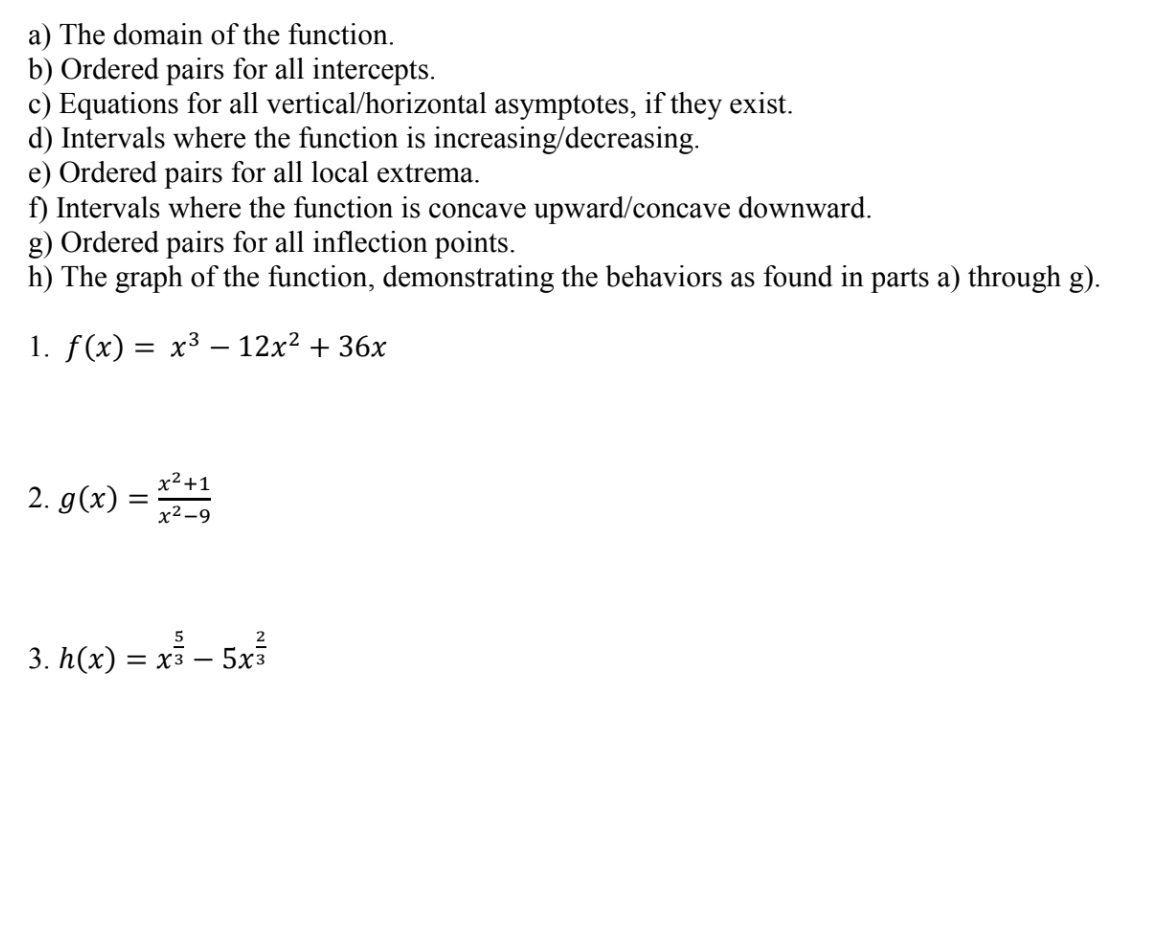# a) The domain of the function.b) Ordered pairs for all intercepts.c) Equations for all vertical/horizontal asymptotes, if they existd) Intervals where the function is increasing/decreasinge) Ordered pairs for all local extremaft) Intervals where the function is concave upward/concave downwardg) Ordered pairs for all inflection points.h) The graph of the function, demonstrating the behaviors as found in parts a) through g)I. f(x)-x3-12x2 + 36X2x2-9523. h(x) -xs - 5x3

Question

Q#3, f to h. I'm having a problem identifying the critical #s for the second derivative of H. I keep landing different answers and this affected my graph. Please clarify the problem for me. Thank youhelp_outlineImage Transcriptionclosea) The domain of the function. b) Ordered pairs for all intercepts. c) Equations for all vertical/horizontal asymptotes, if they exist d) Intervals where the function is increasing/decreasing e) Ordered pairs for all local extrema ft) Intervals where the function is concave upward/concave downward g) Ordered pairs for all inflection points. h) The graph of the function, demonstrating the behaviors as found in parts a) through g) I. f(x)-x3-12x2 + 3 6X 2 x2-9 5 2 3. h(x) -xs - 5x3 fullscreen
check_circleExpert Solution
Step 1

Note: For subpart problems, we help with only 3 subparts. But since you requested help on understanding critical points and how they would affect the graph, I'll show the work for finding critical values and will provide you the required graph along with answers of all the other sub-parts, if you have any confusion on how an answer to a particular part was obtained you can put up a new request for detailed answers for the parts you would need clarity on.

Step 2

#3:

Critical points are those points where derivative is either zero or undefined. If derivative is undefined at a point, then for that point to be a critical point, the original function must be defined at that point.

As shown below, the critical points for first derivative are x = 0 and x = 2.

Step 3

Similary, we can find critical points for second derivative (we call them potential inflection points) as shown below:

So, we get the potential inflection points (what you refer as critical numbers for second derivative) to be x=-3/2 and x = 0.

But when we check the si...

### Want to see the full answer?

See Solution

#### Want to see this answer and more?

Solutions are written by subject experts who are available 24/7. Questions are typically answered within 1 hour*

See Solution
*Response times may vary by subject and question
Tagged in

### Derivative